# For which of the mixtures will ag2so4(s) precipitate?

150.0 mL of 0.10 M Na2SO4(aq) and 5.0
mL of 0.20 M AgNO3(aq)
150.0 mL of 0.10 M Na2SO4(aq) and 5.0 mL of 0.30 M AgNO3(aq)
150.0 mL of 0.10 M Na2SO4(aq) and 5.0 mL of 0.40 M AgNO3(aq)
150.0 mL of 0.10 M Na2SO4(aq) and 5.0 mL of 0.50 M
AgNO3(aq)
150.0 mL of 0.10 M Na2SO4(aq) and 5.0
mL of 0.20 M AgNO3(aq)
150.0 mL of 0.10 M Na2SO4(aq) and 5.0 mL of 0.30 M AgNO3(aq)
150.0 mL of 0.10 M Na2SO4(aq) and 5.0 mL of 0.40 M AgNO3(aq)
150.0 mL of 0.10 M Na2SO4(aq) and 5.0 mL of 0.50 M
AgNO3(aq) 150.0 mL of 0.10 M Na2SO4(aq) and 5.0 mL of 0.30 M AgNO3(aq) 150.0 mL of 0.10 M Na2SO4(aq) and 5.0 mL of 0.40 M AgNO3(aq) 150.0 mL of 0.10 M Na2SO4(aq) and 5.0 mL of 0.50 M
AgNO3(aq)

General guidance

Concepts and reason

The solubility product for the reaction is equilibrium constant where the solid ionic compound dissociates into its ions in a solution. The solubility product is denoted as. The solubility product value relates to the saturated solution and indicates the precipitate level of the compound. The formation precipitation starts when ionic product exceeds the solubility product.

Fundamentals

The solubility product value of the compound depends on the concentrations of its ions in a solution.
Example: AB is a solid ionic compound.

Precipitation: If the solubility product value is lesser than the concentration of the ions present in the solution, the compound precipitates in the solution.

Step-by-step

Step 1 of 5

The given solid ionic compound is
The equilibrium equation for the ionic compound is given below:

Therefore, the solubility product of the ionic compound is

The equilibrium equation has been written for the given solid ionic compound , and the solubility product has been derived from the equilibrium equation.

Take care while writing the solubility product of the given solid ionic compound.
Correct:

Incorrect:

Identify the possibility of the formation of the precipitate from the mixture using the solubility product.

Step 2 of 5

The balanced equation for the reaction of

The initial concentration of ions in is given below:

The initial concentration of ions in is given below:

The ionic product of is given below:

The ionic product is less than the solubility product ,

For the mixture, precipitation does not take place.

The balanced equation for the reaction of is given in which the two moles of react with one mole of to produce the precipitate. The concentration of silver ions and sulphate ions has been calculated to find the ionic product. The value of ionic product is less than that of the solubility product for which the precipitation does not takes place.

Do not get confused while calculating the ionic product of
Correct:

Incorrect:

Identify the possibility of formation of the precipitate from the mixture using the solubility product.

Step 3 of 5

The balanced equation for the reaction of

The initial concentration of ions in is given below:

The initial concentration of ions in is given below:

The ionic product of is given below:

The ionic product is less than the solubility product,

For the mixture, precipitation does not take place.

The concentration of silver ions and sulphate ions has been calculated to find the ionic product. The value of ionic product is less than that of the solubility product for which precipitation does not takes place.

Identify the possibility of formation of precipitate from the mixture using solubility product.

Step 4 of 5

The balanced equation for the reaction of

The initial concentration of ions in is given below:

The initial concentration of ions in is given below:

The ionic product of is given below:

The ionic product is greater than the solubility product ,

For the mixture, precipitation takes place.

The concentration of silver ions and sulphate ions has been calculated to find the ionic product. The value of ionic product is greater than that of the solubility product; therefore, the precipitation does take place.

Identify the possibility of the formation of precipitate from the mixture using the solubility product.

Step 5 of 5

The balanced equation for the reaction of

The initial concentration of ions in is given below:

The initial concentration of ions in is given below:

The ionic product of is given below:

The ionic product is greater than the solubility product,

For the mixture, precipitation takes place.

The balanced equation for the reaction of is given in which the two moles of react with one mole of to produce the precipitate. The concentration of silver ions and sulphate ions has been calculated to find the ionic product. The value of ionic product is greater than that of the solubility product for which the precipitation does take place.

For the mixture, precipitation does not take place.

For the mixture, precipitation does not take place.

For the mixture, precipitation takes place.

For the mixture, precipitation takes place.

For the mixture, precipitation does not take place.

For the mixture, precipitation does not take place.

For the mixture, precipitation takes place.

For the mixture, precipitation takes place.

AB(s) A+ (aq) +B* (aq) K. =[A+][B’]
Ag,SO
Ag,SO (s) 2Ag+ (aq)+S0; (aq)
K-=[Ag*) [so?] = 1.2×10-5
(Ag,SO)
K.=[g*][so]
K. =[Ag*][so;-
Ag,SO
150.0mL of 0.10 M Na,SO, (aq) and 5.0 mL of 0.20 M AgNO, (aq)
AgNO, and Na2SO,
2AgNO, (aq) + Na,0(aq) → Ag,SO, (aq)+NaNO, (aq)
[agt
AgNO3
[Ag* uitian = 5.Oml0.20mmol AgNO, 1 mol Agt mL *1 mol AgNO, (150.0+5.0)mL = 0.00645M
[SO]
Na,SO
[soz) = 150.0ml.x 0.10mmol Na So 1 mol O2- mL “1 mol Na,so, (150.0+5.0)mL = 0.0968M
Ag,SO
K, =[ag*.. [SO ) =(0.00645)’ (0.0968) = 4.0×106
K,
AgNO, and Na,so
AgNO3
Na,SO
Ag,SO
[agt
[SO]
Ag,SO
K, =[ag*.. [SO ) =(0.00645)’ (0.0968) = 4.0×106
“[os] [„Sv]=”y =(0.00645)(0.0968) = 6.04×10-5
Ag,SO
150.0mL of 0.10 M Na So. (aq) and 5.0 mL of 0.30 M AgNO, (aq)
AgNO, and Na2SO,
2AgNO, (aq) + Na,0(aq) → Ag,SO, (aq)+NaNO, (aq)
[agt
AgNO3
[Ag* uitian = 50ml 0.30mmol AgNO, 1 mol Agt mL *1 mol AgNO, (150.0+5.0)mL = 0.00968M
[SO]
Na,SO
[soz) = 150.0ml.x 0.10mmol Na So 1 mol O2- mL “1 mol Na,so, (150.0+5.0)mL = 0.0968M
Ag,SO
9-01*16= (89600),(896000) **[os]”][ .sv]=””>
K,
[agt
[SO]
Ag,SO
150.0mL of 0.10 M Na,SO, (aq) and 5.0 mL of 0.40 M AgNO, (aq)
AgNO, and Na2SO,
2AgNO, (aq) + Na,0(aq) → Ag,SO, (aq)+NaNO, (aq)
[agt
AgNO3
[Ag* uition = 50ml. 0.40mmol AgNO, 1 mol Agt mL 1 mol AgNO, (150.0+5.0)mL = 0.0129M
[SO]
Na,SO
[soz) = 150.0ml.x 0.10mmol Na So 1 mol O2- mL “1 mol Na,so, (150.0+5.0)mL = 0.0968M
Ag,SO
K,” =[Ag+ Titang (so? Justin = (0.0129)’ (0.0968) = 1.6×10-5
K, >K, → precipitation takes place
[agt
[SO]
Ag,SO
150.0mL of 0.10 M Na,So, (aq) and 5.0 mL of 0.50 M AgNO, (aq)
AgNO, and Na2SO,
2AgNO, (aq) + Na,0(aq) → Ag,SO, (aq)+NaNO, (aq)
[agt
AgNO3
[Ag* uition = 50ml. 0.50mmol AgNO, 1 mol Agt mL 1 mol AgNO, (150.0+5.0)mL = 0.0161M
[SO]
Na,SO
[soz) = 150.0ml.x 0.10mmol Na So 1 mol O2- mL “1 mol Na,so, (150.0+5.0)mL = 0.0968M
Ag,SO
K,” =[Ag*i [so? Julia =(0.0161) (0.0968) = 2.5×10-5
K, >K, → precipitation takes place
AgNO, and Na,so
AgNO3
Na,SO
Ag,SO
[agt
[SO]
150.0mL of 0.10 M Na,SO, (aq) and 5.0 mL of 0.20 M AgNO, (aq)
150.0mL of 0.10 M Na,SO, (aq) and 5.0 mL of 0.20 M AgNO, (aq)
150.0mL of 0.10 M Na,SO, (aq) and 5.0 mL of 0.20 M AgNO, (aq)
150.0mL of 0.10 M Na SO,(aq)
5.0 mL of 0.20 M AgNO, (aq)
150.0mL of 0.10 M Na So. (aq) and 5.0 mL of 0.30 M AgNO, (aq)
150.0mL of 0.10 M Na So. (aq) and 5.0 mL of 0.30 M AgNO, (aq)
150.0mL of 0.10 M Na So. (aq) and 5.0 mL of 0.30 M AgNO, (aq)
We were unable to transcribe this image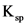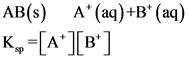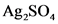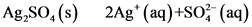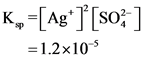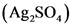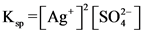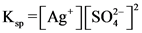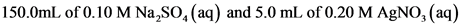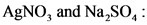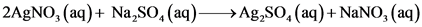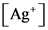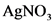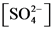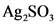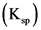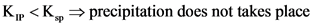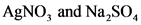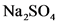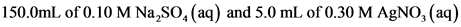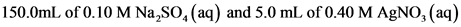This is the collective voice of the talented editorial team at Answerprime.com. Committed to delivering high-quality content, this account represents the collaborative efforts of knowledgeable experts. With a passion for research and a dedication to accuracy, Answerprime brings you insightful articles across various subjects. Explore a diverse range of expertise and perspectives curated by the editorial team through the lens of Answerprime.
Latest posts by Answer Prime (see all)
Scroll to Top